# How to Calculate and Solve for Horizontal Strain | Rock Mechanics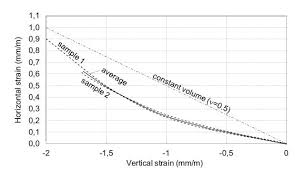The image above represents horizontal strain.

To compute for horizontal strain, five essential parameters are needed and these parameters are Principal Horizontal Stress Component 1 (σH1), Principal Horizontal Stress Component 2 (σH2), Poisson’s Ratio (v), Vertical Stress (σv) and Young’s Modulus (E).

The formula for calculating the horizontal strain:

εH = H1 – vσH2 – vσv) / E

Where:

εH = Horizontal Strain
σH1 = Principal Horizontal Stress Component 1
σH2 = Principal Horizontal Stress Component 2
v = Poisson’s Ratio
σv = Vertical Stress
E = Young’s Modulus

Let’s solve an example;
Find the horizontal strain when the principal horizontal stress component 1 is 7, the principal horizontal component 2 is 9, the Poisson’s ratio is 11, the vertical stress is 21 and young’s modulus is 27.

This implies that;

σH1 = Principal Horizontal Stress Component 1 = 7
σH2 = Principal Horizontal Stress Component 2 = 9
v = Poisson’s Ratio = 11
σv = Vertical Stress = 21
E = Young’s Modulus = 27

εH = H1 – vσH2 – vσv) / E
εH = (7 – 11(9) – 11(21)) / 27
εH = (7 – 99 – 231) / 27
εH = -323 / 27
εH = -11.96

Therefore, the horizontal strain is -11.96.

Calculating the Principal Horizontal Stress Component 1 when the Horizontal Stress, Principal Horizontal Stress Component 2, Poisson’s Ratio, Vertical Stress and Young’s Modulus is Given.

σH1 = (εH x E) + vσH2 + vσv

Where;

σH1 = Principal Horizontal Stress Component 1
εH = Horizontal Strain
σH2 = Principal Horizontal Stress Component 2
v = Poisson’s Ratio
σv = Vertical Stress
E = Young’s Modulus

Let’s solve an example;
Find the principal horizontal stress component 1 when the horizontal strain is 22, the principal horizontal component 2 is 10, the Poisson’s ratio is 7, the vertical stress is 12 and young’s modulus is 3.

This implies that;

εH = Horizontal Strain = 22
σH2 = Principal Horizontal Stress Component 2 = 10
v = Poisson’s Ratio = 7
σv = Vertical Stress = 12
E = Young’s Modulus = 3

σH1 = (εH x E) + vσH2 + vσv
σH1 = (22 x 3) + 7(10) + 7(12)
σH1 = 66 + 70 + 84
σH1 = 220

Therefore, the principal horizontal stress component 1 is 220.

Calculating the Principal Horizontal Stress Component 2 when the Horizontal Stress, Principal Horizontal Stress Component 1, Poisson’s Ratio, Vertical Stress and Young’s Modulus is Given.

σH2 = -(H x E) – σH1 + vσv / v)

Where;

σH2 = Principal Horizontal Stress Component 2
εH = Horizontal Strain
σH1 = Principal Horizontal Stress Component 1
v = Poisson’s Ratio
σv = Vertical Stress
E = Young’s Modulus

Let’s solve an example;
Find the principal horizontal stress component 2 when the horizontal strain is 28, the principal horizontal component 1 is 14, the Poisson’s ratio is 5, the vertical stress is 17 and young’s modulus is 7.

This implies that;

εH = Horizontal Strain =28
σH1 = Principal Horizontal Stress Component 1 = 14
v = Poisson’s Ratio = 5
σv = Vertical Stress = 17
E = Young’s Modulus = 7

σH2 = -(H x E) – σH1 + vσv / v)
σH2 = -((28 x 7) – 14 + 5(17) / 5)
σH2 = -(196 – 14 + 85 / 5)
σH2 = -(182 + 85 / 5)
σH2 = -(267 / 5)
σH2 = -(53.4)
σH2 = -53.4

Therefore, the principal horizontal stress component 2 is -53.4.

Calculating the Poisson’s Ratio when the Horizontal Stress, Principal Horizontal Stress Component 1, Principal Horizontal Stress Component 2, Vertical Stress and Young’s Modulus is Given.

v = σH1 – (εH x E) / σH1 + σH2

Where:

v = Poisson’s Ratio
εH = Horizontal Strain
σH1 = Principal Horizontal Stress Component 1
σH2 = Principal Horizontal Stress Component 2
σv = Vertical Stress
E = Young’s Modulus

Let’s solve an example;
Find the Poisson’s ratio when the principal horizontal stress component 1 is 2, the principal horizontal component 2 is 4, the horizontal strain is 19, the vertical stress is 26 and young’s modulus is 12.

This implies that;

εH = Horizontal Strain = 19
σH1 = Principal Horizontal Stress Component 1 = 2
σH2 = Principal Horizontal Stress Component 2 = 4
σv = Vertical Stress = 26
E = Young’s Modulus = 12

v = σH1 – (εH x E) / σH1 + σH2
v = 2 – (19 x 12) / 2 + 4
v = 2 – 228 / 6
v = -226 / 6
v = -37.6

Therefore, the Poisson’s ratio is -37.6.

Calculating the Vertical Stress when the Horizontal Stress, Principal Horizontal Stress Component 1, Principal Horizontal Stress Component 2, Poisson’s Ratio and Young’s Modulus is Given.

σv = -(H x E) – σH1 + vσH2 / v)

Where:

σv = Vertical Stress
εH = Horizontal Strain
σH1 = Principal Horizontal Stress Component 1
σH2 = Principal Horizontal Stress Component 2
v = Poisson’s Ratio
E = Young’s Modulus

Let’s solve an example;
Find the vertical stress when the horizontal strain is 27, the principal horizontal stress component 1 is 12, the principal horizontal component 2 is 9, the Poisson’s ratio is 24 and young’s modulus is 8.

This implies that;

εH = Horizontal Strain = 27
σH1 = Principal Horizontal Stress Component 1 = 12
σH2 = Principal Horizontal Stress Component 2 = 9
v = Poisson’s Ratio = 24
E = Young’s Modulus = 8

σv = -(H x E) – σH1 + vσH2 / v)
σv = -((27 x 8) – 12 + 8(9) / 24)
σv = -(216 – 12 + 72 / 24)
σv = -(204 + 72 / 24)
σv = -(276 / 24)
σv = -(11.5)
σv = -11.5

Therefore, the vertical stress is -11.5.

Calculating the Young’s Modulus when the Horizontal Stress, Principal Horizontal Stress Component 1, Principal Horizontal Stress Component 2, Poisson’s Ratio and Vertical Stress is Given.

E = H1 – vσH2 – vσv) / εH

Where:

E = Young’s Modulus
εH = Horizontal Strain
σH1 = Principal Horizontal Stress Component 1
σH2 = Principal Horizontal Stress Component 2
v = Poisson’s Ratio
σv = Vertical Stress

Let’s solve an example;
Find the young’s modulus when the horizontal strain is 44, principal horizontal stress component 1 is 28, the principal horizontal component 2 is 5, the Poisson’s ratio is 4 and the vertical stress is 3.

This implies that;

εH = Horizontal Strain = 44
σH1 = Principal Horizontal Stress Component 1 = 28
σH2 = Principal Horizontal Stress Component 2 = 5
v = Poisson’s Ratio = 4
σv = Vertical Stress = 3

E = H1 – vσH2 – vσv) / εH
E = (28 – 4(5) – 4(3)) / 44
E = (28 – 20 – 12) / 44
E = (8 – 12) / 44
E = (-4) / 44
E = -0.09

Therefore, the young’s modulus is -0.09.

Nickzom Calculator – The Calculator Encyclopedia is capable of calculating the horizontal strain.

To get the answer and workings of the horizontal strain using the Nickzom Calculator – The Calculator Encyclopedia. First, you need to obtain the app.

You can get this app via any of these means:

To get access to the professional version via web, you need to register and subscribe for NGN 1,500 per annum to have utter access to all functionalities.
You can also try the demo version via https://www.nickzom.org/calculator

Apple (Paid) – https://itunes.apple.com/us/app/nickzom-calculator/id1331162702?mt=8
Once, you have obtained the calculator encyclopedia app, proceed to the Calculator Map, then click on Geology under Add-on.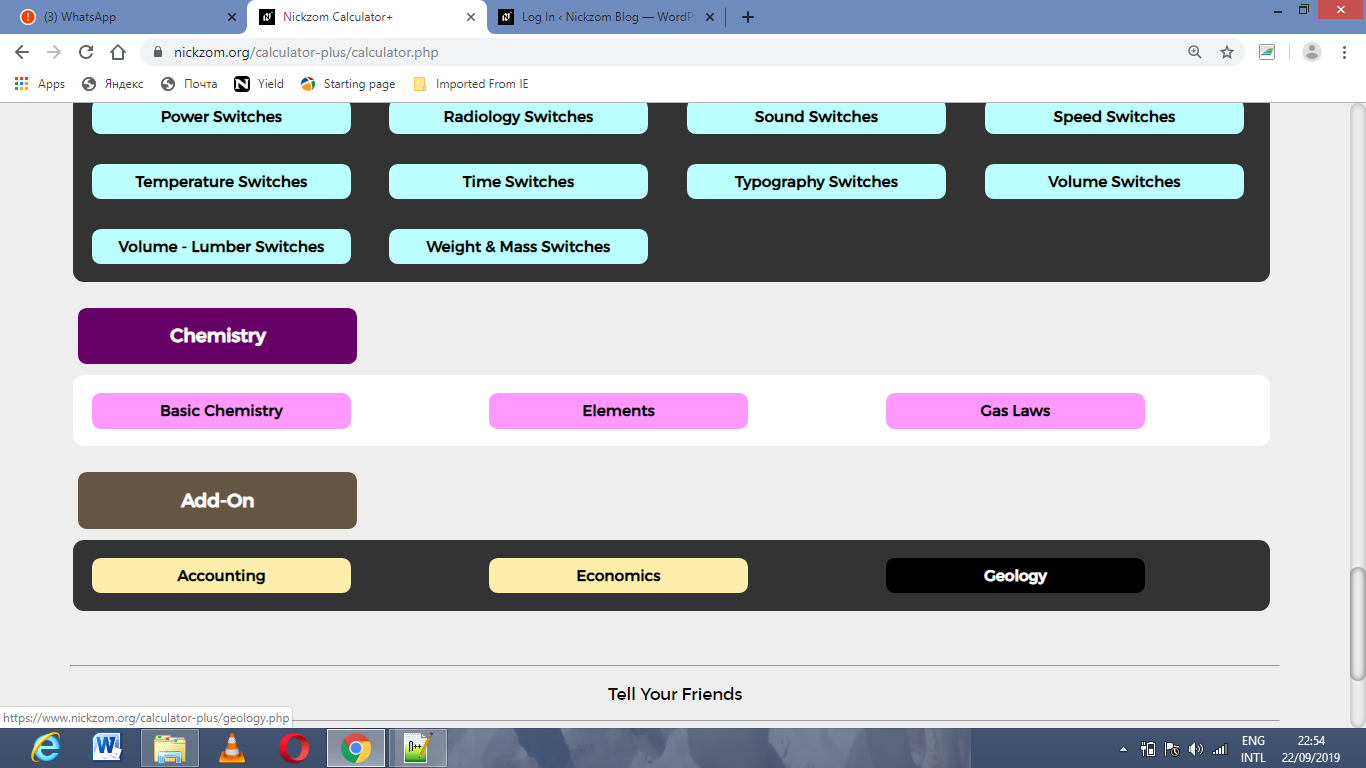Now, Click on Rock Mechanics under GeologyNow, Click on Horizontal Strain under Rock MechanicsThe screenshot below displays the page or activity to enter your values, to get the answer for the horizontal strain according to the respective parameters which are the Principal Horizontal Stress Component 1 (σH1), Principal Horizontal Stress Component 2 (σH2), Poisson’s Ratio (v), Vertical Stress (σv) and Young’s Modulus (E).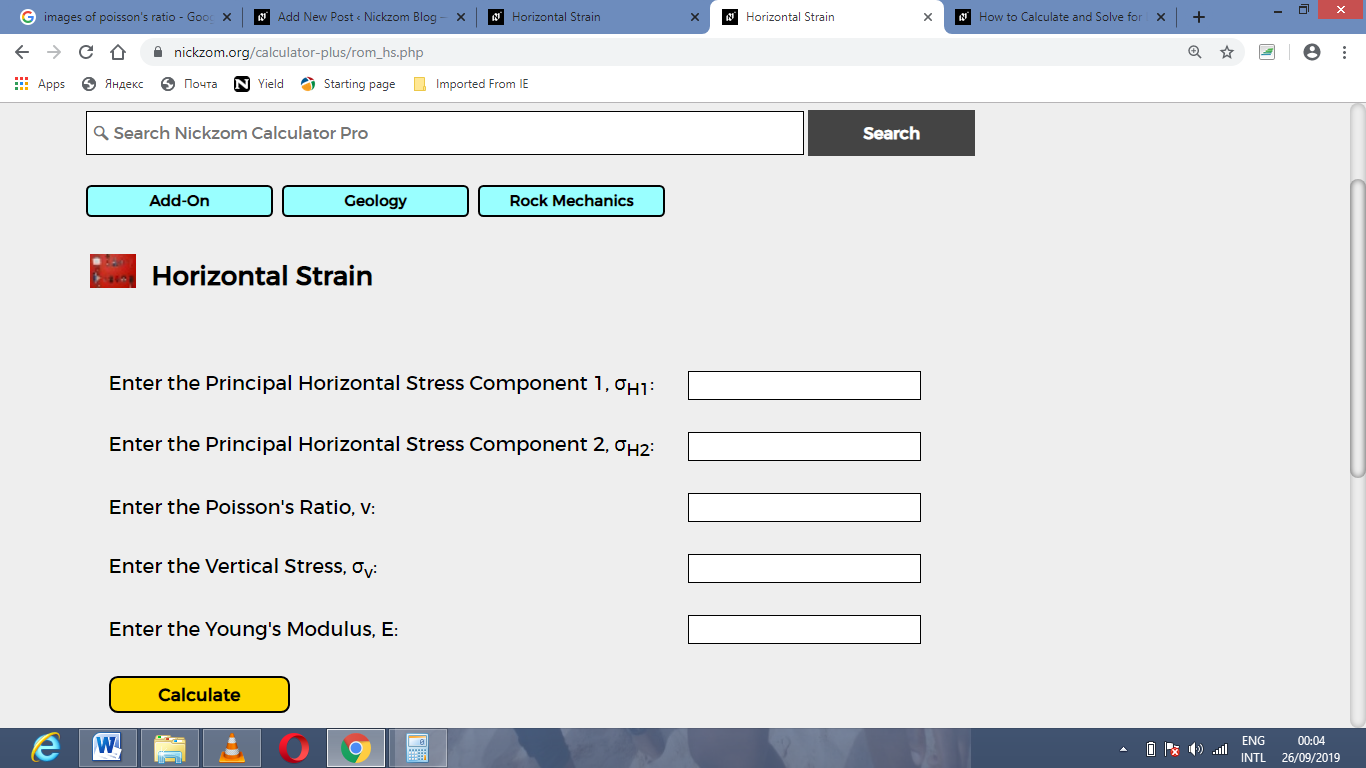Now, enter the values appropriately and accordingly for the parameters as required by the Principal Horizontal Stress Component 1 (σH1) is 7, Principal Horizontal Stress Component 2 (σH2) is 9, Poisson’s Ratio (v) is 11, Vertical Stress (σv) is 21 and Young’s Modulus (E) is 27.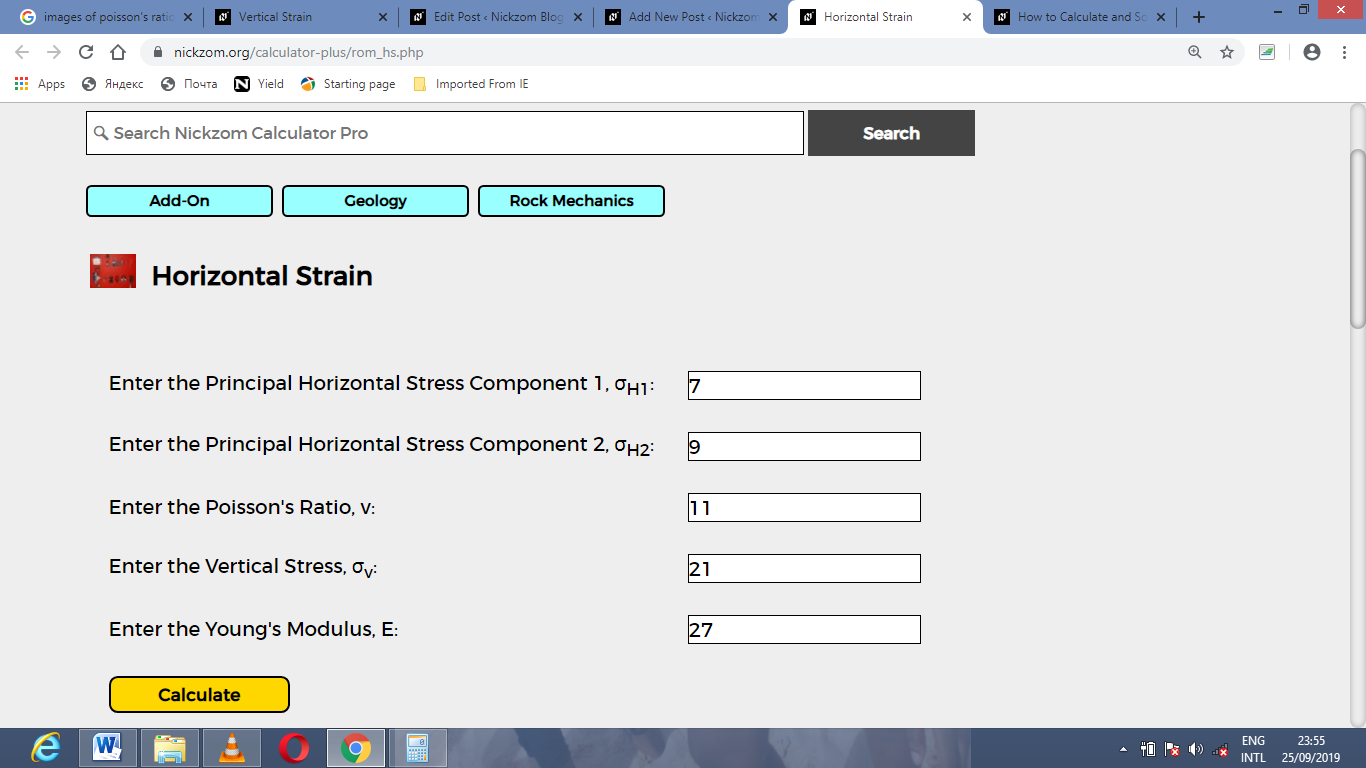Finally, Click on Calculate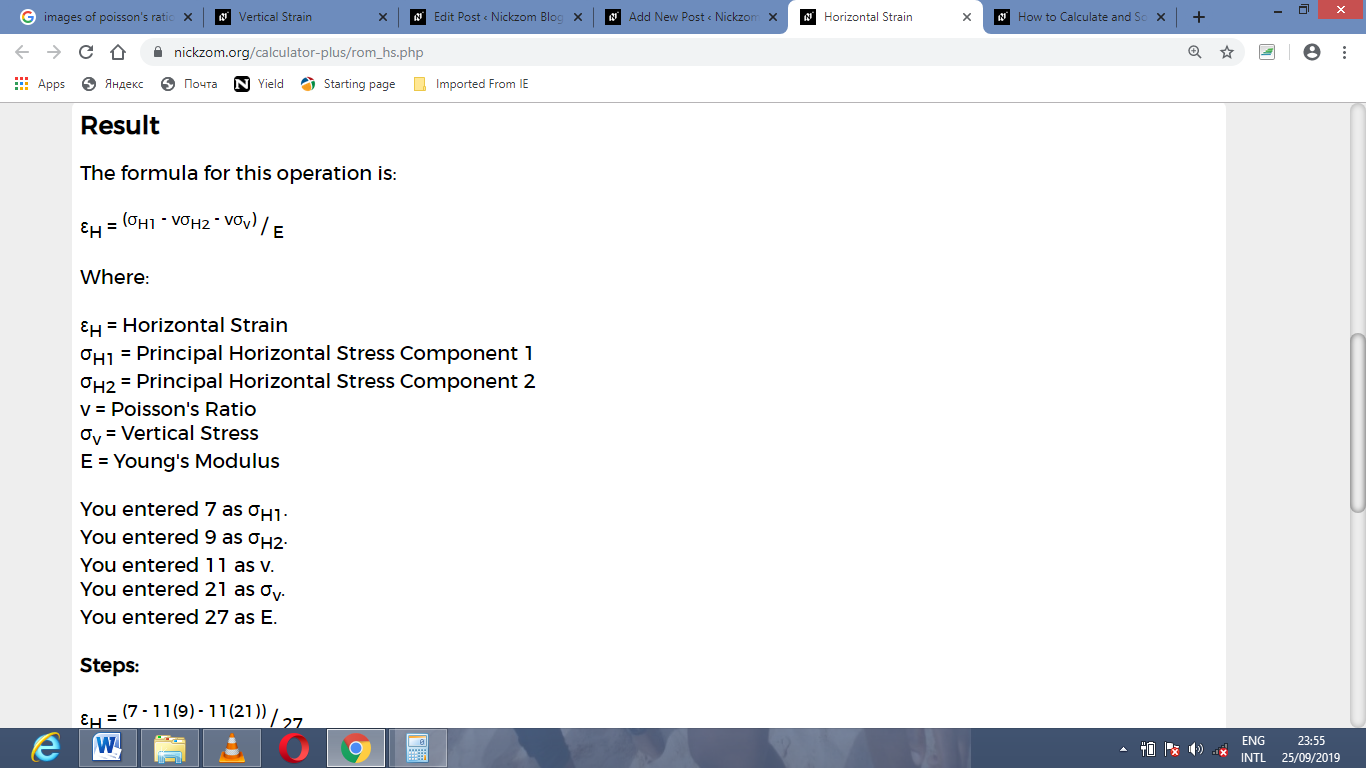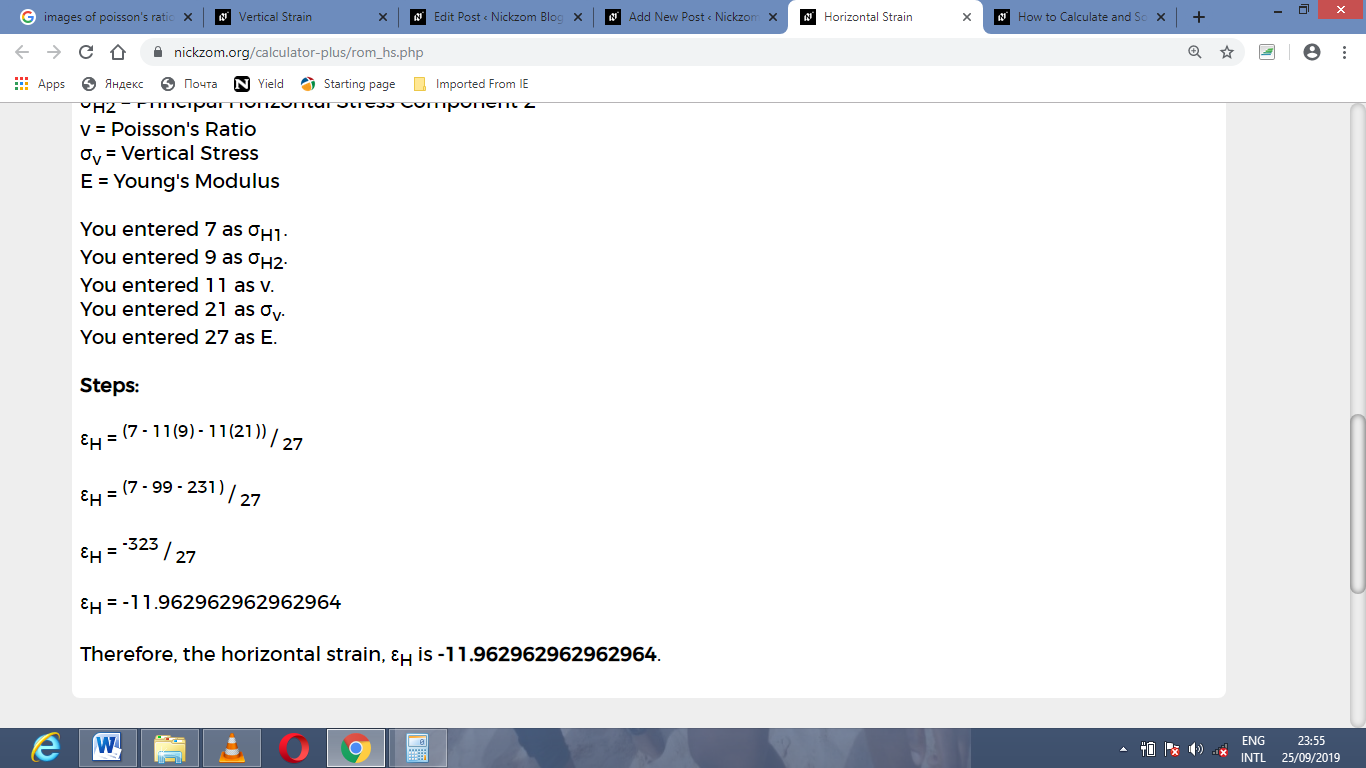As you can see from the screenshot above, Nickzom Calculator– The Calculator Encyclopedia solves for the horizontal strain and presents the formula, workings and steps too.